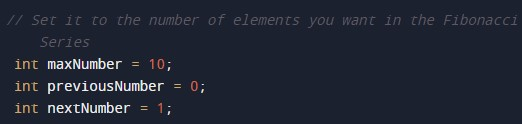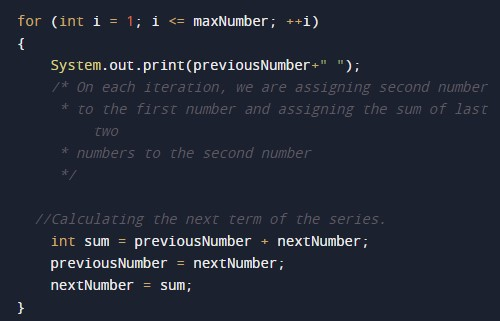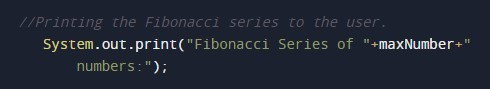# Java Program to Generate Fibonacci Series using Recursion

In this tutorial you will learn about the Java Program to Generate Fibonacci Series using Recursion and its application with practical example.

## Java Program to Generate Fibonacci Series using Recursion

In this tutorial, we will learn to create a Java program that will Print the Fibonacci Series Program using Java programming.

## Prerequisites

Before starting with this tutorial, we assume that you are the best aware of the following Java programming topics:

• Operators in Java Programming.
• Basic Input and Output function in Java Programming.
• Basic Java programming.
• For loop in Java Programming.
• Functions in Java Programming.

## What is Fibonacci Series?

The Fibonacci series is a series that is strongly related to Binet’s formula (golden ratio). In the Fibonacci series, the series starts from 0, 1 and then the third value is generated by adding those numbers.

## Programs to Generate Fibonacci Series:-

In this program, we take the number of elements for the series from the user. Then we will generate the Fibonacci series using the while loop. At last, we will print the series using the print function. The code for the program is given below.

## Program:-

Program to Generate Fibonacci Series.

## Output:-In the above program, we have first initialized the required variable• i = it will hold the integer value for the loop.

Generating the Fibonacci series using the loops.Program Code for Printing the series.In this tutorial we have learn about the Java Program to Generate Fibonacci Series using Recursion and its application with practical example. I hope you will like this tutorial.